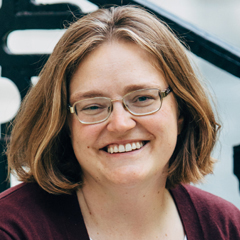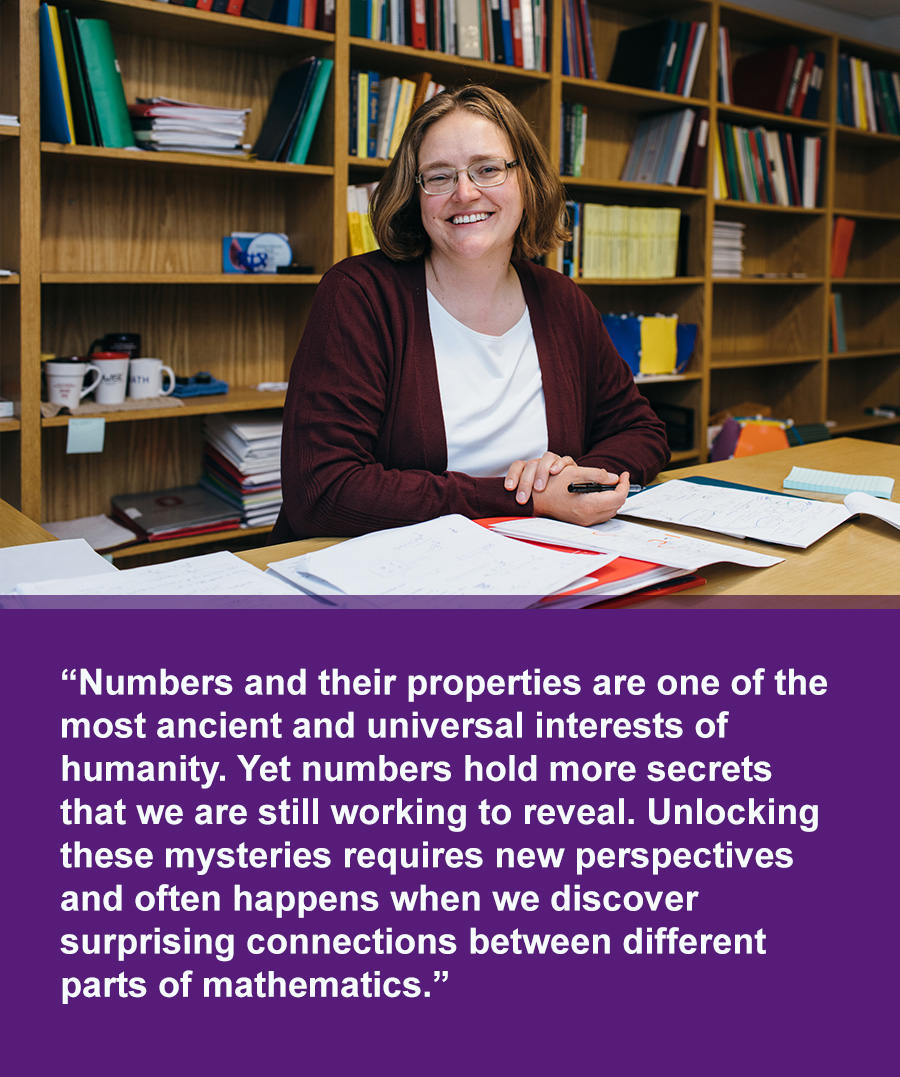Search# Melanie Matchett Wood

## Mathematician | Class of 2022

Addressing foundational questions in number theory from the perspective of arithmetic statistics.Photos for download > Download video file >
Title
Mathematician
Affiliation
Department of Mathematics, Harvard University
Location
Cambridge, Massachusetts
Age
41 at time of award
Area of Focus
Website
Published September 26, 2022

Melanie Matchett Wood is a mathematician investigating foundational questions in pure mathematics. She specializes in number theory (the properties and relationships of whole numbers) and algebraic geometry (the study of geometric structures, such as curves and surfaces, that are described using polynomial equations). By combining a breadth of mathematical approaches, she reveals new ways to see fundamental properties of numbers.

Wood’s research is often motivated by questions in arithmetic statistics. Much of mathematics research focuses on developing proofs for conjectures, but in some cases, it is not feasible to check that a hypothesis is true for all possible cases. For example, determining some properties of prime numbers relies on the behavior of infinitely many numbers (such is the case, for example, for the twin prime conjecture, which posits that there are an infinite number of prime numbers that are 2 apart, such as 11 and 13). Wood takes an arithmetic statistics approach to such problems. She develops probabilistic models for number theoretic objects (such as prime numbers) to reveal how they will behave on average. Wood and collaborators developed such a model for the distribution of ranks of elliptic curves. The rank is a measurement that conveys information about how many of the solutions to that curve’s equation are rational numbers. Curves with higher ranks have larger and more complicated sets of rational solutions. It has been previously assumed that rank is unbounded, meaning it should be possible to find curves with arbitrarily high ranks. However, Wood and her collaborators predicted that there are a finite number of elliptic curves with rank greater than 21, making the set of ranks of elliptic curves over the rational numbers bounded.

In other work, Wood introduced new techniques to determine the behavior of sandpile groups (models of dynamical systems that self organize around a critical point) of random graphs. And her research on the Cohen-Lenstra heuristics (a set of conjectures about the distribution of class groups of quadratic number fields) has advanced understanding of the structure of these groups and for more general families of situations. Wood is revealing new properties of natural numbers that are relevant to other mathematical conjectures and theorems, thereby setting the stage for new discoveries in number theory in the future.

## Biography

Melanie Matchett Wood received a BS (2003) from Duke University, a Certificate of Advanced Study in Mathematics (2004) from the University of Cambridge, and a PhD (2009) from Princeton University. She was a researcher with the American Institute of Mathematics (2009–2017) and held faculty positions at Stanford University (2009–2011), the University of Wisconsin at Madison (2011–2019), and the University of California at Berkeley (2019–2020). In 2020, she became a professor at Harvard University and Radcliffe Alumnae Professor at the Radcliffe Institute for Advanced Study. Wood has published in a variety of leading journals, including Inventiones Mathematicae, Journal of the American Mathematical Society, Duke Mathematical Journal, and Journal of the European Mathematical Society.

## In Melanie's Words”Numbers and their properties are one of the most ancient and universal interests of humanity. Yet numbers hold more secrets that we are still working to reveal. Unlocking these mysteries requires new perspectives and often happens when we discover surprising connections between different parts of mathematics.”

Select News Coverage of Melanie Matchett Wood

High-resolution photos of MacArthur Fellows are available for download (right click and save), including use by media, in accordance with this copyright policy.

Please credit: John D. and Catherine T. MacArthur Foundation

More Fellows

View All 2022 Fellows

Stay Informed